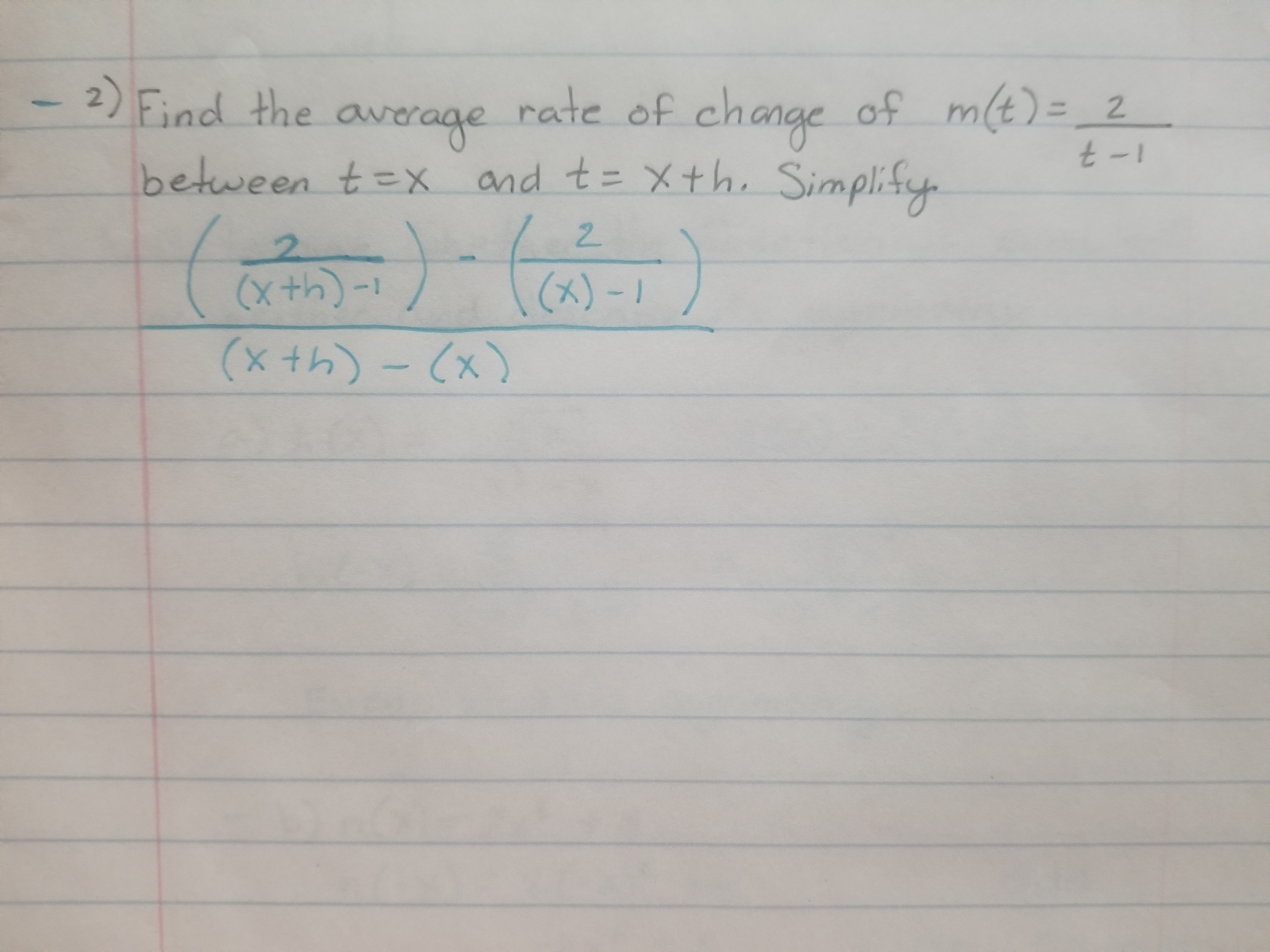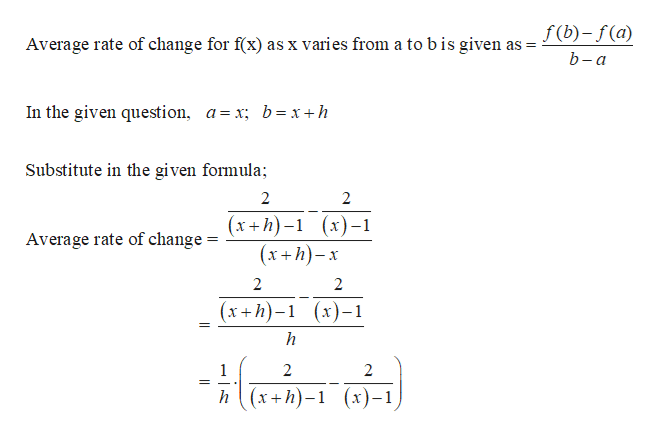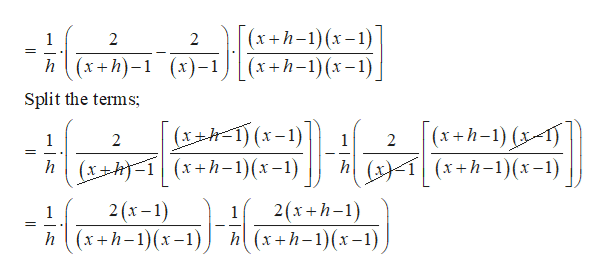# 2 Find the average rate of change of mt)- 2between tex and t xth. Simplifyof ch2(xth)(x)-(xth)-x

Question

I need help working this problem. I get stuck at this point. I think my algebra is failing with the double division. Thank you for any help.help_outlineImage Transcriptionclose2 Find the average rate of change of mt)- 2 between tex and t xth. Simplify of ch 2 (xth) (x)- (xth)-x fullscreen
check_circle

star
star
star
star
star
1 Rating
Step 1

Given function: m(t)=2/(t-1)help_outlineImage Transcriptionclosef(b)-f(a) Average rate of change for f(x) as x varies from a tobis given as b-а In the given question, a=x; b=x +h Substitute in the given formula 2 (х+h)-1 (х)-1 (xh)x Average rate of change = 2 2 (х+h)-1 (х)-1 h - लाच कन) 2 2 (х+h)-1 (х)-1 h fullscreen
Step 2

Mulitply and divide the expression by (x+h-1)(x-1);help_outlineImage Transcriptionclose(х+h-1) (х-1) (х+h-1) (х-1) 1 2 _ = n (x+h)-1 (х)-1 Split the terms; (XH1(x-) hxh (x+h-1)(x-1) (x+h-1) -) 4 (x+h-1)(x-1) 1 2 1 2 h 2 (х -1) h (x+h-1)(х-1) 2(x+h-1) h (x+h-1)(х-1) 1 1 fullscreen
Step 3

Since common denominator has been ow obtained, the ter...

### Want to see the full answer?

See Solution

#### Want to see this answer and more?

Solutions are written by subject experts who are available 24/7. Questions are typically answered within 1 hour.*

See Solution
*Response times may vary by subject and question.
Tagged in

### Functions